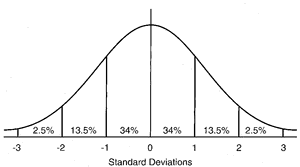# normal distribution

(redirected from Cumulative normal)
Also found in: Dictionary, Thesaurus, Financial, Encyclopedia.

## distribution

[dis″trĭ-bu´shun]
1. the specific location or arrangement of continuing or successive objects or events in space or time.
2. the extent of a ramifying structure such as an artery or nerve and its branches.
3. the geographical range of an organism or disease.
frequency distribution in statistics, a mathematical function that describes the distribution of measurements on a scale for a specific population.
normal distribution a symmetrical distribution of scores with the majority concentrated around the mean; for example, that representing a large number of independent random events. It is in the shape of a bell-shaped curve. Called also gaussian distribution. See illustration.Normal distribution. The approximate percentage of the area (or frequency) lying under the curve between standard deviations is indicated. From Dorland's, 2000.
probability distribution a mathematical function that assigns to each measurable event in a sample group the probability that the event will occur.
Miller-Keane Encyclopedia and Dictionary of Medicine, Nursing, and Allied Health, Seventh Edition. © 2003 by Saunders, an imprint of Elsevier, Inc. All rights reserved.

## nor·mal dis·tri·bu·tion

a specific bell-shaped frequency distribution commonly assumed by statisticians to represent the infinite population of measurements from which a sample has been drawn; characterized by two parameters, the mean (x) and the standard deviation (σ), in the equation:
Farlex Partner Medical Dictionary © Farlex 2012

## normal distribution

A bell-shaped frequency distribution of data, the plotted curve of which is symmetrical about the mean, indicating no significant deviation of the data set from the mean.

Properties of a normal distribution
Continuous and symmetrical, with both tails extending to infinity; arithmetic mean, mode, and median are identical. The curve’s shape is completely determined by the mean and standard deviation.

## gaus·si·an dis·tri·bu·tion

(gow'sē-ăn dis'tri-byū'shŭn)
The statistical distribution of members of a population around the population mean. In a gaussian distribution, 68.2% of values fall within ± 1 standard deviation (SD); 95.4% fall within ± 2 SD of the mean; and 99.7% fall within ± 3 SD of the mean.
Synonym(s): bell-shaped curve, normal distribution.
Medical Dictionary for the Health Professions and Nursing © Farlex 2012

## normal distribution

Gaussian distribution, a distribution which when expressed graphically is bell-shaped. The distribution to which many frequency distributions of biological variables, such as height, weight, intelligence, etc correspond.
Collins Dictionary of Medicine © Robert M. Youngson 2004, 2005

## Gauss,

Johann K.F., German physicist, 1777-1855.
gauss - a unit of magnetic field intensity.
gaussian curve - a specific bell-shaped frequency distribution. Synonym(s): gaussian distribution; normal distribution
gaussian distribution - Synonym(s): gaussian curve

## nor·mal dis·tri·bu·tion

(nōr'măl dis'tri-byū'shŭn)
Specific bell-shaped frequency distribution commonly assumed by statisticians to represent the infinite population of measurements from which a sample has been drawn.
Medical Dictionary for the Dental Professions © Farlex 2012
References in periodicals archive ?
This implies that H([x.sub.3i] [[beta].sub.3]) equals the probability of detecting a malignancy in patient i, conditional upon the patient not actually having such a malignancy, where H([x.sub.3i] [[beta].sub.3]|[x.sub.1i] [[beta].sub.1] + [[epsilon].sub.1] < 0)= H([x.sub.3i] [[beta].sub.3) is the cumulative normal distribution function.
Let F([??]) and H([??]) represent again cumulative normal functions for the malignancy, and detection stage given no malignancy.
) is based on the assumption that seed-survival curves can be accurately described by a negative cumulative normal distribution (Ellis and Roberts, 1980a, b, 1981; Ellis et al., 1982).
Seed survival curves have a symmetrical sigmoid shape that can be described by a negative cumulative normal distribution.

Site: Follow: Share:
Open / Close